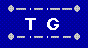Cryptarithms or Alphametics Home  |  Your Feedback  |  Cryptograms

A cryptarithm (or alphametic) is an encoded arithmetic problem. Each digit has been replaced by a letter. The object is to determine the original numbers.

This applet solves simple addition and multiplication cryptarithms, such as this famous one created by Henry Dudeney in 1924:

```    S E N D         9 5 6 7
+   M O R E     +   1 0 8 5
= M O N E Y     = 1 0 6 5 2
```

Enter three "words" in the three upper boxes, choose + for addition or * for multiplication, and hit "Calculate" to see if substituting a different digit for each letter makes it a valid problem.

To enter the problem shown above, you would put send in the first box, more in the second box, and money in the third box. Do not include any blanks or any other characters.

 Ver 15.07.1 + * =